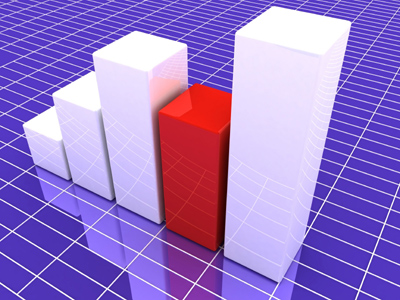Graphs are useful for recording data.

# Level 7-8 Algebra - Graphs 02

Fed up of algebra? Sick of graphs? We have some great news for you! This is the last KS3 Maths quiz in this series on graphs. But there's some bad news too - it tests you on some of the more complicated aspects in the world of graphs.

When it comes to graphs, you'll need to know an exponent from a coordinate. You'll have to work out the steepest and shallowest gradients. You'll be expected to plot graphs using formulas given to you and predict their shape. If you are not yet sure what to do then you've come to the right place. Here you'll get plenty of practise on the harder parts of graphs.

In the following quiz, take your time and read each question carefully before choosing your answers. And don't forget the helpful comments after each question. They can help to clarify anything you aren't sure of. Go on, give it a whirl and see how much you have learned so far.

1.
On a distance-time graph what does a line segment that is horizontal tell you?
The data is incorrect
The distance travelled was slowing down
The distance travelled was speeding up
There was no movement during the period
The line on the time axis continues but on the distance axis it has paused
2.
If a graph equation contains an exponent then what could you definitely say about the line?
It is very long
It is very short
It is straight
It is curved
Remember an exponent is the small number to the upper-right of another number. As soon as you see one of these in an equation you should expect a curve
3.
Two coordinates of a line are as follows: (2, 15) and (6, 10). How might the line best be described?
It is horizontal
It is vertical
It is worth remembering that you can have both positive and negative gradients in ANY quadrant
4.
Which of these equations would give the steepest gradient?
y = x
y = x + 1
y = x + 2
All the above are the same gradient
If all the lines were plotted on the same graph they would run parallel to each other
5.
Which of these equations would give the steepest gradient?
y = x
y = 2x
y = 3x
y = 4x
In the correct answer, each movement of a unit along the x axis means a movement of 4 units up the y axis
6.
Two coordinates of a line are as follows: (3, 12) and (2, 8). What is the equation?
y = 2x
y = 4x
y = 6x
y = 8x
If you plot the coordinates you will see that for every unit moved along the x axis, you move 4 units up the y axis. Working out an equation in this way has the grand name of the 'gradient-intercept' method
7.
Which of these graphs would give you the most shallow gradient?
y = 3x - 1
y = 4x - 1
y = 5x - 1
y = 6x -1
The more times you multiply x, the steeper the gradient becomes
8.
What is the mid point of the line (1, 9) (5, 15)
(1, 15)
(3, 12)
(6, 24)
(9, 15)
Take the first number in the first bracket and add it to the first number in the second bracket and divide the answer by two - gives you 3. Then do the same for the second numbers in the brackets and you will get 12
9.
Two coordinates of a line are as follows: (3, 21) and (2, 14). How might the line best be described?
It is horizontal
It is vertical
Lines that slope from the bottom left to the top right have a positive gradient. If you cannot imagine a line then plot it to see what it looks like
10.
If a graph equation contains only the signs of plus, minus and equals then what could you definitely say about the line?
It is very long
It is very short
It is straight
It is curved
These are the simplest equations to plot